Electron. J. Differential Equations, Vol. 2017 (2017), No. 151, pp. 1-21.

### Nontrivial solutions of inclusions involving perturbed maximal monotone operators Dhruba R. Adhikari

Abstract:
Let X be a real reflexive Banach space and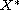its dual space. Let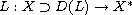be a densely defined linear maximal monotone operator, and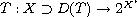,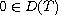and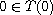, be strongly quasibounded maximal monotone and positively homogeneous of degree 1. Also, let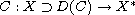be bounded, demicontinuous and of type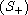w.r.t. to D(L). The existence of nonzero solutions of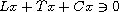is established in the set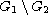, where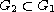with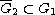,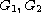are open sets in X,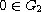, and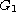is bounded. In the special case when L=0, a mapping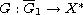of class (P) introduced by Hu and Papageorgiou is also incorporated and the existence of nonzero solutions of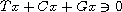, where T is only maximal monotone and positively homogeneous of degree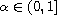, is obtained. Applications to elliptic partial differential equations involving p-Laplacian with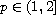and time-dependent parabolic partial differential equations on cylindrical domains are presented.

Submitted June 11, 2016. Published June 25, 2017.
Math Subject Classifications: 47H14, 47H05, 47H11.
Key Words: Strong quasiboundedness; Browder and Skrypnik degree theories; maximal monotone operator; bounded demicontinuous operator of type.

Show me the PDF file (319 KB), TEX file for this article.Dhruba R. Adhikari Department of Mathematics Kennesaw State University Georgia 30060, USA email: dadhikar@kennesaw.edu